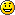#MIDIbox

### Site Tools

fadercore_-_vlr-8x16ledmeter

### Table of Contents

Standard 220mm wide modules

## VLR-8x16LEDmeter

A solution to add 8 Meters with 16 LEDs for each Meter. It contains 2 different Types of PCBs one is the LEDmatrix and the other a slim PCB to mount 16LEDs called meterboard.

### BOM

BIll of Material

Allways look for the cheapest seller, the Price may vary heavy!!! And look for Local dealers preferred. http://www.midibox.org/dokuwiki/doku.php?id=where_to_order_components

To make a small and easy to connect matrix over a hole Frontplatte is no fun. Thats why i made myself a better solution. Based on the common DOUT modules it can be connected to 3 SRs.

##### The Materials: Mouser
• 3x10pin Header, 517-30310-6002
• 6x10pin Sockets, 517-D89110-0131HK
• 16x20pin Header, 517-30320-6002
• 16x20pin Sockets, 517-D89120-0131HK
• 20 Wire Ribbon Cable, 523-135-2801-020FT
• about 80 Green LEDs, 604-WP3A8GD
• about 80 Yellow LEDs, 604-WP3A8YD
• about 80 Red LEDs, 604-WP3A8HD

Project Order Basket on Mouser: http://www.mouser.com/ProjectManager/ProjectDetail.aspx?AccessID=5ecf7b4949

The PCB is available under: https://www.vlrlab.com/home/18-ledmatrix.html https://www.vlrlab.com/home/19-meterboard.html

### How to get Started

The LEDs are mounted to the Edge of the Meterboard to get a very slim form. That makes it a bit harder to solder them but i guess that no one wont be able to do it. To solder the Header to the Edge there is a wite triangle printed to the PCB on one side. This Triange has to match with the Triangle on the Header.

### Prepare the PCB

Well you should now by now how to solder Headers…### Prepare the Connection

Connect the SEL, Row1-8 & Row9-16 with each a DOUT Header.

### Test it in MIOS

I use a Encoder connected directly to a DIN to test. Assuming that the DOUT is the first device on the chain of J8/9 we need to configure it like this:

RESET_HW

LCD “%C”
LCD “@(1:1:1)OLED1”

# Test D-OUT LEDmatrix board

DOUT_MATRIX n=1 rows=8 inverted=1 sr_dout_sel1=1 sr_dout_r1=2 sr_dout_r2=3

# Matrix control by a Encoder connected to DIN
ENC n=1 sr=1 pins=0:1 type=detented2

EVENT_ENC id= 1 fwd_id=LED_MATRIX:1 type=CC chn= 1 cc= 24 lcd_pos=1:1:2 label=“^std_enc” LED_MATRIX_PATTERN=1

LED_MATRIX_PATTERN n= 1 pos= 0 pattern=0000000000000000
LED_MATRIX_PATTERN n= 1 pos= 1 pattern=1000000000000000
LED_MATRIX_PATTERN n= 1 pos= 2 pattern=1100000000000000
LED_MATRIX_PATTERN n= 1 pos= 3 pattern=1110000000000000
LED_MATRIX_PATTERN n= 1 pos= 4 pattern=1111000000000000
LED_MATRIX_PATTERN n= 1 pos= 5 pattern=1111100000000000
LED_MATRIX_PATTERN n= 1 pos= 6 pattern=1111110000000000
LED_MATRIX_PATTERN n= 1 pos= 7 pattern=1111111000000000
LED_MATRIX_PATTERN n= 1 pos= M pattern=1111111100000000
LED_MATRIX_PATTERN n= 1 pos= 8 pattern=1111111110000000
LED_MATRIX_PATTERN n= 1 pos= 9 pattern=1111111111000000
LED_MATRIX_PATTERN n= 1 pos=10 pattern=1111111111100000
LED_MATRIX_PATTERN n= 1 pos=11 pattern=1111111111110000
LED_MATRIX_PATTERN n= 1 pos=12 pattern=1111111111111000
LED_MATRIX_PATTERN n= 1 pos=13 pattern=1111111111111100
LED_MATRIX_PATTERN n= 1 pos=14 pattern=1111111111111110
LED_MATRIX_PATTERN n= 1 pos=15 pattern=1111111111111111

Im using my VLR-8oDisp board to show the Values of the encoder. You can change it to any other type of Display-setting… lcd_pos=6:1:5 {6=Display number : 1= X-axis : 5= Y-Axis (row)}

For any Comment or Question: Forum Thread???

fadercore_-_vlr-8x16ledmeter.txt · Last modified: 2014/07/30 11:58 by novski

### Page Tools